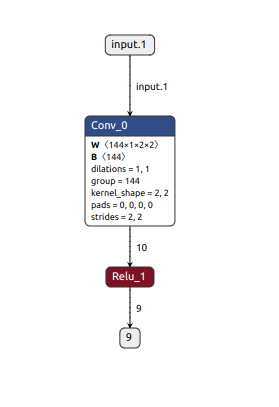# CBUF size limit calculation

Continuing the discussion from Fail at runing conv layer on DLA:

Hi, I went through the previous post but I’m still facing a bit of confusion regarding how those values are being calculated.

`roundUp(numChannels * kernelHeight * kernelWidth * 32, 128) / (cbufEntryWidth * cbufEntriesPerBank)`
Specifically, in the above statement how do we get the value for `cbufEntryWidth` and `cbufEntriesPerBank`.

I’m attaching an example of a single layer model that fails to run on the dla. Can you please explain the formula here and use this new onnx as an example to show how it is exceeding the CBUF limit of 16.
The warning I get: [W] [TRT] Default DLA is enabled but layer Conv_x is not supported on DLA, falling back to GPU.onnx file:
test_single_layer_2.onnx (5.9 KB)

System:

• NVIDIA Jetson Xavier NX (Developer Kit Version)
• Jetpack 4.6 [L4T 32.6.1]
• NV Power Mode: MODE_20W_6CORE - Type: 8
• jetson_stats.service: active
• Libraries:
• CUDA: 10.2.300
• cuDNN: 8.2.1.32
• TensorRT: 8.0.1.6
• Visionworks: 1.6.0.501
• OpenCV: 4.4.0 compiled CUDA: YES
• VPI: ii libnvvpi1 1.1.12 arm64 NVIDIA Vision Programming Interface library
• Vulkan: 1.2.70

NOTE: I need to use TensorRT version 8.0.1.6 and cannot upgrade it. Please provide the explanation for this version.

Hi,

We have added this information in the future TensorRT release.
So you will get the details about CBUF calculation when deploying with DLA.

Thanks.

Sadly things are still unclear to me. If I use `bufEntryWidth=128` and `cbufEntriesPerBank=256` then,

``````import numpy as np

INT8 = False
inputDims_c = 144
inputDims_w = 144
kernelSize_h = 3
kernelSize_w = 3
dilation_h = 1

entriesPerDataSlice = np.ceil(np.ceil(inputDims_c * (1 if INT8 else 2) / 32.0) * inputDims_w /4.0).astype(np.uint32)
dilatedKernelHt = (kernelSize_h - 1) * dilation_h + 1

wtBanksForOneKernel = np.ceil(
np.round(inputDims_c * kernelSize_h * kernelSize_w * (1 if INT8 else 2) * 32, 128) / (128.0*256.0)).astype(np.uint32)
minDataBanks = np.ceil(float(entriesPerDataSlice * dilatedKernelHt) / 256.0).astype(np.uint32)

print(entriesPerDataSlice) # 324
print(dilatedKernelHt) # 3
print(wtBanksForOneKernel) # 3
print(minDataBanks) # 4
``````

So, as per the documentation this value is less than 16 and should thus run on dla. I think I’m making some error in the way I’m trying to calculate the value.

I’d be grateful if you could show me how the value of `wtBanksForOneKernel` and `minDataBanks` are calculated.

Also, I tried by reducing the kernel size and that onnx worked. Attaching the new onnx as well.
test_single_layer_2x2.onnx (3.1 KB)Hi,

We are checking this internally.

Thanks.

Hi,

Here is the CUBUF log output for the first (failure) model:

``````[11/25/2022-02:15:37] [W] [TRT] CBUF validation failed because the total number of weight and data banks exceeds the maximum allotted number of banks.
Number of weight banks
= roundUp(numChannels * kernelHeight * kernelWidth * 32, 128) / (cbufEntryWidth * cbufEntriesPerBank)
= roundUp(144 * 3 * 3 * 32, 128) / (128 * 256)
= 2
Number of data banks
= (entriesPerDataSlice * dilatedKernelHeight) / cbufEntriesPerBank
= (1512 * 3) / 256
= 18,
where:
entriesPerDataSlice
= ceil(ceil(numChannels * bytesPerElement / 32) * kernelWidth / 4)
= ceil(ceil(144 * 2 / 32) * 3 / 4)
= 1512
Maximum allotted banks = 16, which is less than 2 + 18.
``````

Thanks.

1 Like

Hi,

Thanks a lot for the reply. I feel like I’m missing something here:
144 * 2 = 288.
288/32 = 9.
ceil(9) = 9

9 * 3 = 27
27/4 = 6.75
ceil(6.75) = 7

so,
`ceil(ceil(144 * 2 / 32) * 3 / 4)`
= `ceil(ceil(9) * 3 / 4)`
= `ceil( 27 / 4)`
= `7`

by this formula, we are still within limits.

Hi,

We are double-checking this with our internal team.

Thanks.

Hi,

There is a typo in the output log that the kernelWidth should be inputWidth.
So the entriesPerDataSlice calculation should be:

``````entriesPerDataSlice
= ceil(ceil(numChannels * bytesPerElement / 32) * inputWidth / 4)
= ceil(ceil(144 * 2 / 32) * 672 / 4)
= 1512
``````

We have fixed this in our internal branch.
Sorry for the confusion.

Thanks.

1 Like

This topic was automatically closed 14 days after the last reply. New replies are no longer allowed.